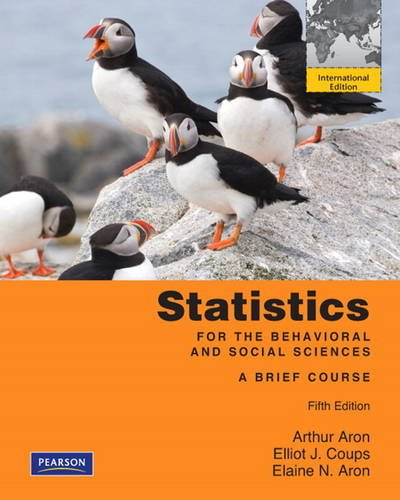### Statistics for The Behavioral and Social Sciences:A Brief Course: International Edition

 Yazar : Arthur Aron ; Elliot Coups ; Elaine N. Aron Yayınevi : Pearson Education ISBN : 9780205008605 Baskı yılı : 2010 Sayfa sayısı : 504 Edisyon : 5 Stok durumu : Tükendi

For one-quarter/semester courses that focus on the basics in statistics or combine statistics with research methods.

?

The?fifth edition of Statistics for the Behavioral and Social Sciences builds off an already well-established?approach - emphasizing the intuitive, deemphasizing the mathematical, and explaining everything in direct, simple language - but also goes beyond these principles to further student understanding. By using?definitional formulas to emphasize the concepts of?statistics, rather than rote memorization, students work problems in a way that keeps them constantly aware of the underlying logic of?what they are doing.?

Chapter 1?- Displaying the Order in a Group of Numbers Using Tables and Graphs

?

Chapter 2 - The Mean, Variance, Standard Deviation, and Z Scores

?

Chapter 3?- Correlation and Prediction

?

Chapter 4?- Some Key Ingredients for Inferential Statistics: The Normal Curve, Sample Versus Population, and Probability

?

Chapter 5?- Introduction to Hypothesis Testing

?

Chapter 6?- Hypothesis Tests with Means of Samples

?

Chapter 7?- Making Sense of Statistical Significance: Effect Size and Statistical Power

?

Chapter 8?- Introduction to the t Test: Single Sample and Dependent Means

?

Chapter 9?- The t Test for Independent Means

?

Chapter 10 - Introduction to the Analysis of Variance

?

Chapter 11- Chi-Square Tests and Strategies When Population Distributions Are Not Normal

?

Chapter 12?- Applying Statistical Methods in Your Own Research Project